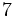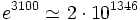# Goldbach's conjecture

Jump to: navigation, search

## Statement

The conjecture has the following equivalent forms:

• Every even integer greater thanis expressible as a sum of two (possibly equal) primes.
• Every even integer greater thanis expressible as a sum of two (possibly equal) odd primes.

A partition of an even integer as a sum of two primes is termed a Goldbach partition.

## Relation with other facts and conjectures

### Weaker conjectures and facts

Name of conjecture/fact Statement Status
Weak Goldbach conjecture every odd integer greater thanis expressible as the sum of three odd primes corollary of generalized Riemann hypothesis
Vinogradov's theorem every sufficiently large odd integer is expressible as the sum of three odd primes. Also finds that there are many such triples. The current bound of sufficiently large is approximatelyproved
Haselgrove's strengthening of Vinogradov's theorem in the statement of Vinogradov's theorem, we can choose the three primes to be roughly equal proved
Chaohua's strengthening of Vinogradov's theorem numerically strengthens Haselgrove's statement proved
Schnirelmann's theorem on Goldbach's conjecture every even integer greater thanis expressible as the sum of at mostprimes proved
Chen's theorem on Goldbach's conjecture every sufficiently large even integer is expressible as the sum of a prime number and a semiprime, i.e., a number that is either prime or is a product of two primes proved